# Future of libraries

You know that thanks to the Internet, electronic communications and developing online resources on the Internet annually declining number of traditional readership by 17%. These deal is irreversible evolutionary shift from old books from libraries to immediately available and cheaper information from the Internet.

The library had in 1991 3336 readers. Determine the year in which count of readers will be only 289.

Correct result:

x =  2004

#### Solution:We would be pleased if you find an error in the word problem, spelling mistakes, or inaccuracies and send it to us. Thank you!Tips to related online calculators

## Next similar math problems:

• Geometric progression 2There is geometric sequence with a1=5.7 and quotient q=-2.5. Calculate a17.
• Six termsFind the first six terms of the sequence a1 = -3, an = 2 * an-1
• Tenth memberCalculate the tenth member of geometric sequence when given: a1=1/2 and q=2
• Terms of GPWhat is the 6th term of the GP 9, 81, 729,. .. ?
• Researchers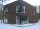Researchers ask 200 families whether or not they were the homeowner and how many cars they had. Their response was homeowner: 14 no car or one car, two or more cars 86, not homeowner: 38 no car or one car, two or more cars 62. What percent of the families
• Five membersWrite first 5 members geometric sequence and determine whether it is increasing or decreasing: a1 = 3 q = -2
• Missing term 2What is the missing term for the Geometric Progression (GP) 3, 15, 75,__, 1875?
• Geometric sequence 4It is given geometric sequence a3 = 7 and a12 = 3. Calculate s23 (= sum of the first 23 members of the sequence).
• A perineumA perineum string is 10% shorter than its original string. The first string is 24, what is the 9th string or term?
• Find next memberFind x if the numbers from a GP 7, 49, x.
• Piano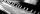If Suzan practicing 10 minutes at Monday; every other day she wants to practice 2 times as much as the previous day, how many hours and minutes will have to practice on Friday?
• Geometric progression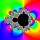Fill 4 numbers between 4 and -12500 to form geometric progression.
• GP - 8 itemsDetermine the first eight members of a geometric progression if a9=512, q=2
• Five element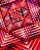The geometric sequence is given by quotient q = 1/2 and the sum of the first six members S6 = 63. Find the fifth element a5.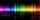The glass of 1 mm thickness absorb 4% of UV radiation passing through. How many percent of UV rays absorb glass with a thickness 1.1 cm, made from 1 mm thick those glasses?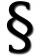Judge JUDr. Usury approved the agreement on guilt and punishment where confessed to pay daily interest 0.18%. How big is a yearly interest? Year has 360 days.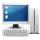The computer was purchased 10000,-. Each year, the price of a computer depreciates always the same percentage of the previous year. After four years, the value of the computer is reduced to 1300,- How many percent was depreciated price of the computer eac